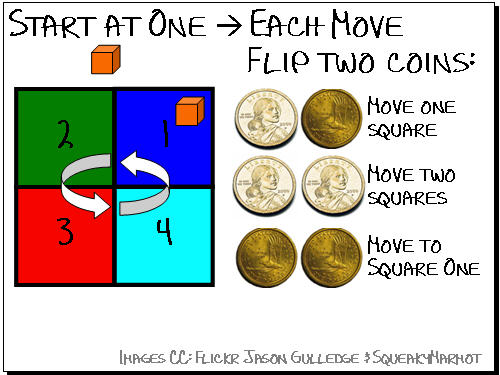# Puzzler: Coin game

This is a simplified version of the first problem I ever attempted to solve in MATLAB. For my original problem, I was trying to figure out the probability of being on any square in the board game Monopoly. That is a difficult problem from a book-keeping sense, but the essence of the problem is in today’s Puzzler.With the rules shown in the diagram, what is the probability of being on each of the four squares after each of the first twenty turns?

I can think of two good ways of solving today’s Puzzler. I look forward to seeing how everyone else does it.

Take a little bit and work on the Puzzler. I don’t want to give away strategies before you get a chance to work on the puzzle, so I am just showing the results of each method in the preview below, but not saying what the method was.

[There is a typo in the comments on this second video. It should say the probability of moving from Square 4 to Square 1, not the other way around. Sorry!]